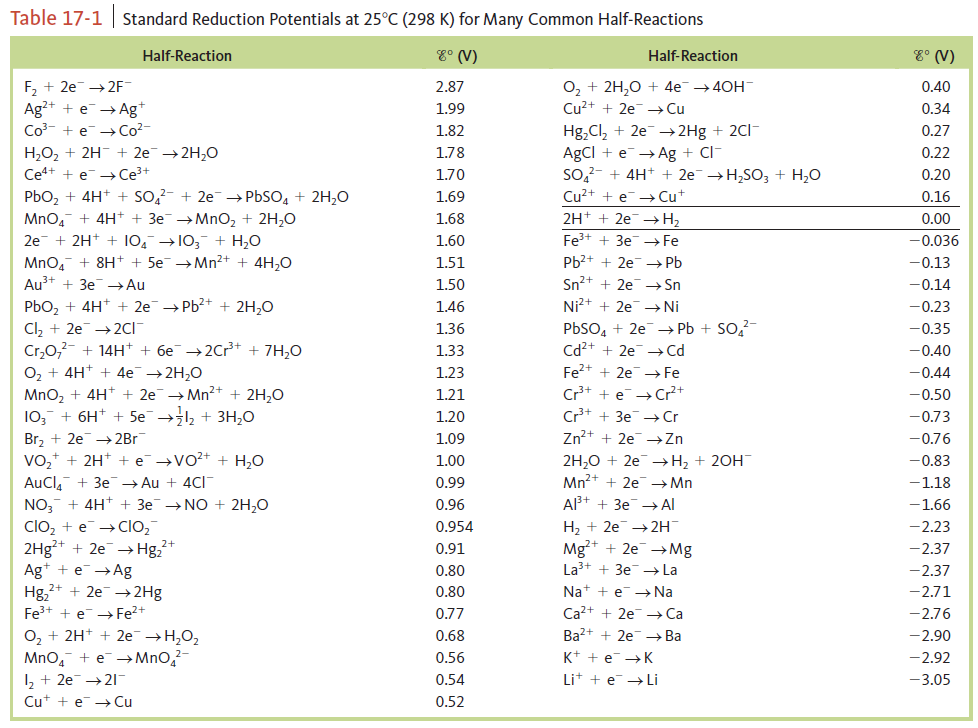# Problem: The saturated calomel electrode, abbreviated SCE, is often used as a reference electrode in making electrochemical measurements. The SCE is composed of mercury in contact with a saturated solution of calomel (Hg2Cl2). The electrolyte solution is saturated KCl. ESCE is +0.242 V relative to the standard hydrogen electrode. Calculate the potential for each of the following galvanic cells containing a saturated calomel electrode and the given half-cell components at standard conditions. In each case, indicate whether the SCE is the cathode or the anode. Standard reduction potentials are found in Table 17‑1.e. Ni2+ + 2e- → Ni

###### FREE Expert Solution

$\overline{){\mathbf{E}}{{\mathbf{°}}}_{{\mathbf{cell}}}{\mathbf{=}}{\mathbf{E}}{{\mathbf{°}}}_{{\mathbf{cathode}}}{\mathbf{-}}{\mathbf{E}}{{\mathbf{°}}}_{{\mathbf{anode}}}}$

E°cell > 0→ spontaneous in the forward direction → cathode > E°anode

ESCE = 0.242 V  → higher → cathode

Ni2+ + 2e- → Ni                     cell = -0.23 V → lower → anode

84% (81 ratings)###### Problem Details

The saturated calomel electrode, abbreviated SCE, is often used as a reference electrode in making electrochemical measurements. The SCE is composed of mercury in contact with a saturated solution of calomel (Hg2Cl2). The electrolyte solution is saturated KCl. ESCE is +0.242 V relative to the standard hydrogen electrode. Calculate the potential for each of the following galvanic cells containing a saturated calomel electrode and the given half-cell components at standard conditions. In each case, indicate whether the SCE is the cathode or the anode. Standard reduction potentials are found in Table 17‑1.

e. Ni2+ + 2e- → Ni